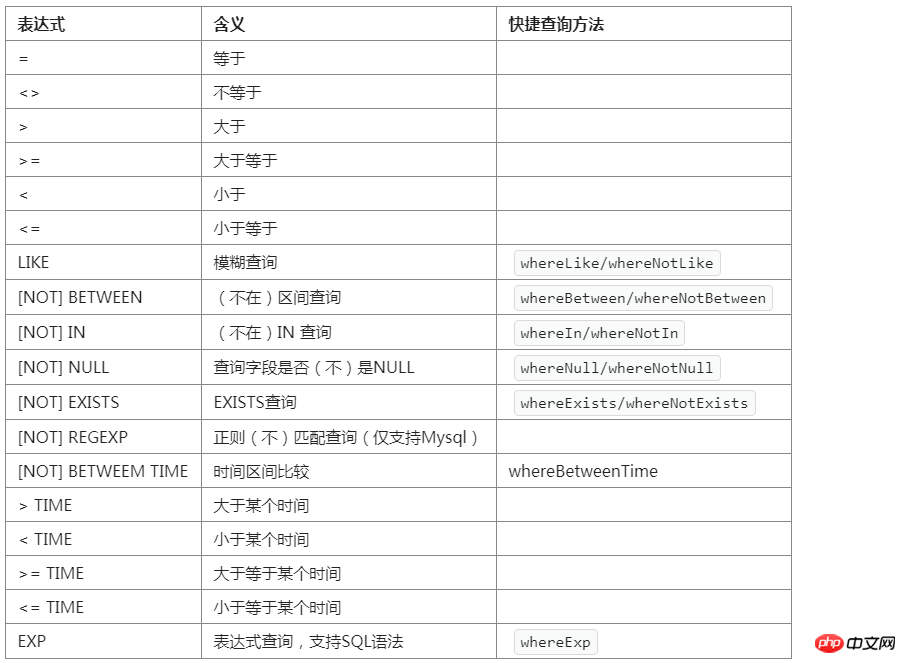﻿ 查询表达式 - [ ThinkPHP5.1完全开发手册 ] - 在线原生手册 - php中文网```where('字段名','表达式','查询条件');
whereOr('字段名','表达式','查询条件');```

5.1还支持新的查询方法

```whereField('表达式','查询条件');
whereOrField('表达式','查询条件');```

Field使用字段的驼峰命名方式。`Db::name('user')->where('id','=',100)->select();`

`Db::name('user')->where('id',100)->select();`

`SELECT * FROM `think_user` WHERE  `id` = 100`

`Db::name('user')->where('id','<>',100)->select();`

`SELECT * FROM `think_user` WHERE  `id` <> 100`

`Db::name('user')->where('id','>',100)->select();`

`SELECT * FROM `think_user` WHERE  `id` > 100`

`Db::name('user')->where('id','>=',100)->select();`

`SELECT * FROM `think_user` WHERE  `id` >= 100`

`Db::name('user')->where('id','<',100)->select();`

`SELECT * FROM `think_user` WHERE  `id` < 100`

`Db::name('user')->where('id','<=',100)->select();`

`SELECT * FROM `think_user` WHERE  `id` <= 100`

[NOT] LIKE： 同sql的LIKE

`Db::name('user')->where('name','like','thinkphp%')->select();`

`SELECT * FROM `think_user` WHERE  `name` LIKE 'thinkphp%'`

like查询支持使用数组

`Db::name('user')->where('name','like',['%think','php%'],'OR')->select();`

`SELECT * FROM `think_user` WHERE  (`name` LIKE '%think' OR `name` LIKE 'php%')`

```Db::name('user')->whereLike('name','thinkphp%')->select();
Db::name('user')->whereNotLike('name','thinkphp%')->select();```

[NOT] BETWEEN ：同sql的[not] between

`Db::name('user')->where('id','between','1,8')->select();`

`Db::name('user')->where('id','between',[1,8])->select();`

`SELECT * FROM `think_user` WHERE  `id` BETWEEN 1 AND 8`

```Db::name('user')->whereBetween('id','1,8')->select();
Db::name('user')->whereNotBetween('id','1,8')->select();```

[NOT] IN： 同sql的[not] in

`Db::name('user')->where('id','in','1,5,8')->select();`

`Db::name('user')->where('id','in',[1,5,8])->select();`

`SELECT * FROM `think_user` WHERE  `id` IN (1,5,8)`

```Db::name('user')->whereIn('id','1,5,8')->select();
Db::name('user')->whereNotIn('id','1,5,8')->select();```

[NOT] IN查询支持使用闭包方式

[NOT] NULL ：

```Db::name('user')->where('name', null)
->where('email','null')
->where('name','not null')
->select();```

`SELECT * FROM `think_user` WHERE  `name` IS NULL  AND `email` IS NULL  AND `name` IS NOT NULL`

```Db::name('user')->where('title','=', 'null')
->where('name','=', 'not null')
->select();```

```Db::name('user')->whereNull('name')
->whereNull('email')
->whereNotNull('name')
->select();```

EXP：表达式

`Db::name('user')->where('id','in','1,3,8')->select();`

`Db::name('user')->where('id','exp',' IN (1,3,8) ')->select();`

exp查询的条件不会被当成字符串，所以后面的查询条件可以使用任何SQL支持的语法，包括使用函数和字段名称。

`Db::name('user')->where('id','>=',100)->select();`

`Db::name('user')->whereId('>=',100)->select();`